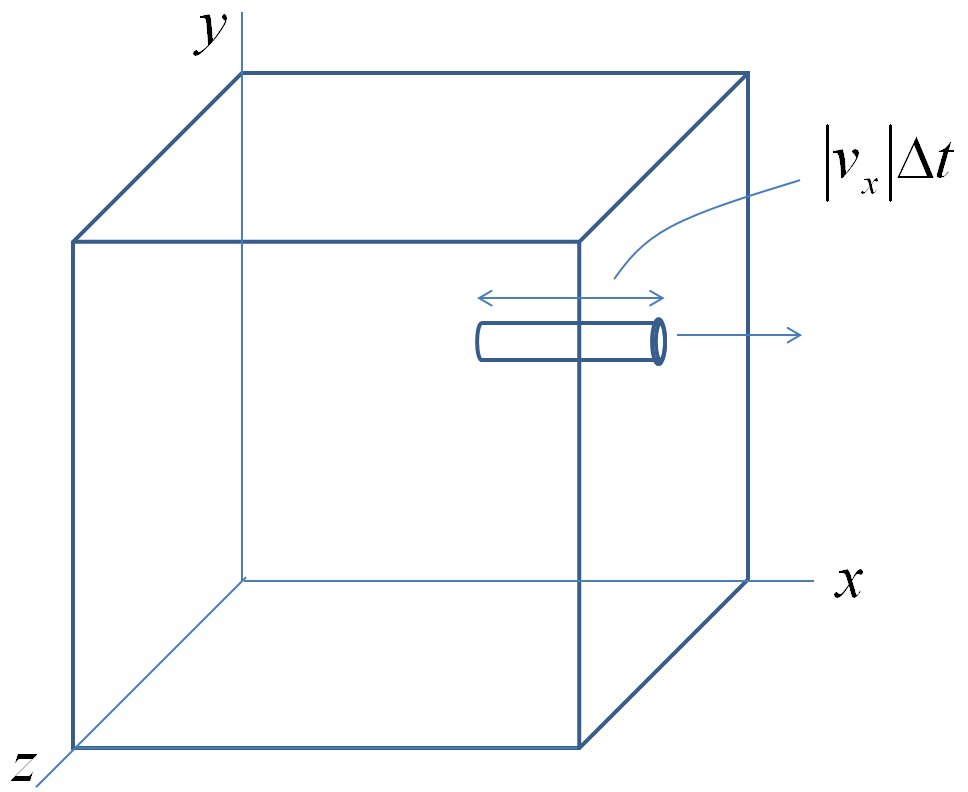## Section27.5Effusion

Effusion is process where a gas exits from a container through a tiny hole. Ideally the hole should be much smaller than the mean free path so that the molecules do not suffer collisions among themselves when exiting.

Effusion is used to study the average speed of molecules in the container. According to Graham's law, the average kinetic energies of molecules of different gases at the same temperature are identical.

Average kinetic energy of two gases at the same temperature is same.

This will give the following equality between the kinetic energies of molecules of two gases.

\begin{equation} \frac{1}{2}m_av_a^2 = \frac{1}{2}m_bv_b^2\ \ \text{(same temperature)}\tag{27.5.1} \end{equation}

Therefore, the ratio of their speed will have to be inversely proportional to the square root of their molecular masses.

\begin{equation} \frac{v_a}{v_b}= \sqrt{\frac{m_b}{m_a}}\ \ \text{(same temperature)}\tag{27.5.2} \end{equation}

From this, we can conclude that hydrogen gas in a balloon will effuse $4$ times faster than oxygen and $\sqrt{2}$ times helium.

A container of volume 2 L contains 0.3 moles of Helium gas kept at constant temperature 300 K. There is a small hole of area$0.003\text{ mm}^2$ though which gas molecules escape. The density of molecules outside is so low that no molecules get back into the container. Find the pressure inside the container after 0.2 seconds. Assume all the atoms arriving at the hole leave the container.

Hint

Use the rms-speed for the escape speed and set up an equation for the rate of decrease of mass inside the container. From the mass remaining at the end, compute the pressure.

$3.6\ \text{atm}\text{.}$

Solution

We will first find the density of the gas inside the container as a function of time. Let us consider the container in the shape of a cube. Consider a hole of area $A$ in the face perpendicular to the $x$-axis.

The molecules in the volume $|v_x| A \Delta t$ with the $x$-component of velocity positive will eject the hole in time $\Delta t\text{.}$ We have found that we can estimate the magnitude of $v_x$ from the root-mean square speed.\begin{equation*} |v_x| = \sqrt{\langle v_x^2 \rangle} = \frac{1}{\sqrt{3}} v_\text{rms}. \end{equation*}

As a result the mass of gas will decrease with time. The decrease in mass of gas inside the container will be

\begin{equation*} \Delta m = - \rho \times |v_x| A \Delta t, \end{equation*}

where $\rho$ is the density of the gas at time $t\text{,}$

\begin{equation*} \rho = \frac{m}{V_0}, \end{equation*}

where $V_0$ is the volume of the container. Therefore,

\begin{equation*} \frac{ \Delta m}{\Delta t} = - \frac{ |v_x| A}{V_0} m. \end{equation*}

Taking the infinitesimal limit we obtain the following for the rate of change of mass of the gas inside the container.

\begin{equation} \frac{ d m}{d t} = - \frac{ m}{\tau}, \label{eq-ch21-pr-5-1}\tag{27.5.3} \end{equation}

where the constants in the formula are lumped into $\tau\text{,}$ a constant quantity that has the unit of time.

\begin{equation*} \tau = \frac{V_0}{ |v_x| A} = \frac{\sqrt{3}V_0}{ v_\text{rms} A} . \end{equation*}

The solution of Eq. (27.5.3) is

\begin{equation*} m(t) = M\ e^{-t/\tau}, \end{equation*}

where $M$ is the mass of the gas in the container at time $t=0\text{.}$ To convert this information to the pressure, we will assume that the mechanical equilibrium exists at each instant so that we can assign a value of pressure in the container although the content of the container would be changing with time. The ideal gas equation can be used to relate mass of the gas in the container to the pressure.

\begin{equation*} p V = n RT = \frac{m}{\text{mol. wt.}} RT. \end{equation*}

where mol. wt. is the molecular weight, which for He is 4 g/mol.

\begin{equation*} p = \frac{MRT}{\text{(mol. wt.)} V} \ e^{-t/\tau} = p_0\ e^{-t/\tau}, \end{equation*}

where $p_0$ is the pressure at time $t=0\text{.}$ Now, we can use the numerical values given in the problem.

\begin{align*} \amp p_0 = \frac{0.3\ \text{mol} \times 0.082\ \text{L.atm/mol.K} \times 300\ \text{K}}{2\ \text{L}} = 3.7\ \text{atm}.\\ \amp v_\text{rms} = \sqrt{\frac{3 k_B T}{m_{He}}} = 1370\ \text{m/s}.\\ \amp \tau = \frac{\sqrt{3}V_0}{ v_\text{rms} A} = \frac{\sqrt{3}\times 2\times 10^{-3} \ \text{m}^3}{ 1370\ \text{m/s}\times 3\times 10^{-9}\ \text{m}^2} = 840\ \text{s}. \end{align*}

The pressure at $t=0.4$ s will be (if my numbers are correct)

\begin{equation*} p (t=0.2\ \text{s})= 3.7\ \text{atm}\ e^{-0.2\ \text{s}/840\ \text{s}} \approx 3.7\ \text{atm}. \end{equation*}
\begin{equation*} p (t=20\ \text{s})= 3.7\ \text{atm}\ e^{20\ \text{s}/840\ \text{s}} \approx 3.6\ \text{atm}. \end{equation*}